The QUANTSELECT Procedure (Experimental)

MODEL Statement

MODEL dependent = <effects> / <options> ;

The MODEL statement names the dependent variable and the covariate effects, including covariates, main effects, constructed effects, interactions, and nested effects; see the section Specification of Effects in Chapter 42: The GLM Procedure, for more information. If you omit the explanatory effects, PROC QUANTSELECT fits an intercept-only model.

After the keyword MODEL, specify the dependent (response) variable, followed by an equal sign, followed by the explanatory effects.

Table 78.8 summarizes the options available in the MODEL statement.

Table 78.8: MODEL Statement Options

Option

Description

Specifies the level of effect selection detail to display

HIERARCHY=

Specifies hierarchy of effects to impose

Specifies models without an explicit intercept

Specifies quantile levels to be applied

Specifies effect selection method

Specifies additional statistics to be displayed

Specifies the test type for computing significance levels

The following list provides details about the options that you can specify in the MODEL statement after a slash (/):

DETAILS=ALL | SUMMARY

specifies the level of effect selection detail that is displayed. The DETAILS=SUMMARY option produces only the selection summary table. The DETAILS=ALL option produces the following:

• entry and removal statistics for each variable that is selected in the model building process

• average check loss and parameter estimates

• entry and removal statistics for the top five candidates for inclusion or exclusion at each step

• a selection summary table

The default is DETAILS=SUMMARY.

HIERARCHY=keyword
HIER=keyword

specifies whether and how the model hierarchy requirement is applied. This option also controls whether a single effect or multiple effects are allowed to enter or leave the model in one step. You can specify that only CLASS effects, or both CLASS and continuous effects, be subject to the hierarchy requirement. This option is ignored unless you also specify one of the following options: SELECTION=FORWARD, SELECTION=BACKWARD, or SELECTION=STEPWISE.

Model hierarchy refers to the requirement that for any term to be in the model, all model effects contained in the term must be present in the model. For example, in order for the interaction A*B to enter the model, the main effects A and B must be in the model. Likewise, neither effect A nor effect B can leave the model while the interaction A*B is in the model.

You can specify the following keywords:

NONE

specifies that model hierarchy not be maintained. Any single effect can enter or leave the model at any given step of the selection process.

SINGLE

specifies that only one effect enter or leave the model at one time, subject to the model hierarchy requirement. For example, suppose that the model contains the main effects A and B and the interaction A*B. In the first step of the selection process, either A or B can enter the model. In the second step, the other main effect can enter the model. The interaction effect can enter the model only when both main effects have already entered. Also, before A or B can be removed from the model, the A*B interaction must first be removed. All effects (CLASS and interval) are subject to the hierarchy requirement.

SINGLECLASS

is the same as HIERARCHY=SINGLE except that only CLASS effects are subject to the hierarchy requirement.

The default is HIERARCHY=NONE.

NOINT

suppresses the intercept term that is otherwise included in the model.

QUANTILES=<number-list  |  PROCESS <(option)>>
QUANTILE=<number-list |  PROCESS <(option)>>

specifies the quantile levels for the quantile regression. You can specify any number of quantile levels in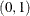. If you do not specify this option, the QUANTSELECT procedure performs median regression effect selection that corresponds to QUANTILES=0.5.

If you specify the QUANTILES=PROCESS option, the QUANTSELECT procedure performs effect selection for quantile process regression. The QUANTILES=PROCESS option cannot be used with LASSO selection methods. You can specify the following option in parentheses after QUANTILES=PROCESS.

NTAU=n  |  ALL

specifies how many quantile levels that you expect to cover for the quantile process. If you specify NTAU=ALL, the QUANTSELECT procedure performs effect selection for accurate quantile process regression. If you specify NTAU=n, the QUANTSELECT procedure performs effect selection for approximate quantile process regression. The approximate quantile process is computed at n equally spaced quantile levels: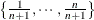.

SELECTION=method <(method-options)>

specifies the method used to select the model, optionally followed by parentheses that enclose method-options that apply to the specified method. The default is SELECTION=STEPWISE.

You can specify the following methods, which are explained in detail in the section Effect Selection Methods.

NONE

specifies full model fitting without effect selection.

FORWARD

specifies forward selection. This method starts with no effects in the model and adds effects.

BACKWARD

specifies backward elimination. This method starts with all effects in the model and deletes effects.

STEPWISE

specifies stepwise regression. This is similar to the FORWARD method except that effects already in the model do not necessarily stay there.

LASSO

specifies a method that adds and deletes parameters based on a version of estimated check risk where the weighted L1-norm of certain weighted regression coefficients is penalized. For more information, see the section LASSO Method (LASSO). If the model contains CLASS variables or constructed effects, these CLASS variables or constructed effects are split into separate covariates.

Table 78.9 lists the applicable method-options for each method.

Table 78.9: Applicable method-options for Each method

method-option

FORWARD

BACKWARD

STEPWISE

LASSO

x

CHOOSE=

x

x

x

x

INCLUDE=

x

x

x

x

MAXSTEP=

x

x

x

x

SELECT=

x

x

x

SLENTRY=

x

x

SLSTAY=

x

x

STOP=

x

x

x

x

STOPHORIZON=

x

x

x

x

You can specify the following method-option in parentheses after the method. As described in Table 78.9, not all method-options apply to every SELECTION= method.

specifies the adaptive LASSO selection method. The ADAPTIVE option can be used only with the SELECTION=LASSO option.

CHOOSE=criterion

chooses from the list of models (with one model at each step of the selection process) the model that yields the best value of the specified criterion as the final selected model. If the optimal value of the specified criterion occurs for more than one model, then the model with the smallest number of parameters is chosen. If you do not specify the CHOOSE= option, then the model selected is the model at the final step in the selection process for the SELECT=SL criterion, or the STOP= option is applied as the CHOOSE= option for all the other cases.

You can specify the following values for criterion in the CHOOSE= option. See the section Criteria Used in Model Selection Methods for more information about these criteria.

chooses the model with the largest adjusted quantile regression R statistic.

AIC

chooses the model with the smallest Akaike’s information criterion.

AICC

chooses the model with the smallest corrected Akaike’s information criterion.

SBC

chooses the model with the smallest Schwarz Bayesian information criterion.

VALIDATE

chooses the model with the smallest average check loss for the validation data. You can specify CHOOSE=VALIDATE only if you have specified a VALDATA= data set in the PROC QUANTSELECT statement or if you have reserved part of the input data for validation by using either a PARTITION statement or a _ROLE_ variable in the input data.

INCLUDE=n

forces the first n effects listed in the MODEL statement to be included in all models. The selection methods are performed on the other effects in the MODEL statement. The INCLUDE= option is available only with SELECTION=FORWARD, SELECTION=STEPWISE, and SELECTION=BACKWARD.

MAXSTEP=n

specifies the maximum number of selection steps. The default value of n is the number of effects in the MODEL statement when SELECTION=FORWARD or SELECTION=BACKWARD and is three times the number of effects when SELECTION=STEPWISE or SELECTION=LASSO.

SELECT=criterion

specifies the criterion that PROC QUANTSELECT uses to determine the order in which effects enter or leave at each step of the specified selection method. This option is not valid when SELECTION=LASSO. You can specify the following values for criterion: ADJR1, AIC, AICC, SBC, SL, and VALIDATE. See the section Criteria Used in Model Selection Methods for more information about these criteria.

When SELECT=SL, the effect selection depends on the selection method and is described in the relevant subsection of the section Effect Selection Methods. Otherwise, the effect that is selected to enter or leave at a step of the selection process is the effect whose addition to or removal from the current model produces the maximum improvement in the specified criterion.

If validation data exist, the default is SELECT=VALIDATE; otherwise, the default is SELECT=SBC.

SLENTRY=value
SLE=value

specifies the significance level for entry, used when the STOP=SL or SELECT=SL option is in effect. The defaults are 0.50 when SELECTION=FORWARD and 0.15 when SELECTION=STEPWISE.

SLSTAY=value
SLS=value

specifies the significance level for staying in the model, used when the STOP=SL or SELECT=SL option is in effect. The defaults are 0.10 when SELECTION=BACKWARD and 0.15 when SELECTION=STEPWISE.

STOP=criterion

specifies the criterion for stopping the selection process. If the maximum number of steps is specified in the MAXSTEP= option and the criterion does not stop the selection process before the maximum number of steps for the selection method, then the selection process terminates at the maximum number of steps.

You can specify the following values for criterion. See the section Criteria Used in Model Selection Methods for more detailed descriptions of these criteria.

NONE

enables the model selection process to go through all possible steps.

stops selection at the step where the next SH= steps (or all remaining steps) would yield models with smaller values of the adjusted quantile regression R (ADJR1) statistic.

AIC

stops selection at the step where the next SH= steps (or all remaining steps) would yield models with larger values of Akaike’s information criterion.

AICC

stops selection at the step where the next SH= steps (or all remaining steps) would yield models with larger values of the corrected Akaike’s information criterion.

SBC

stops selection at the step where the next SH= steps (or all remaining steps) would yield models with larger values of the Schwarz Bayesian information criterion.

VALIDATE

stops selection at the step where the next SH= steps (or all remaining steps) would yield models with larger values of the average check loss for the validation data. You can specify STOP=VALIDATE only if you have specified a VALDATA= data set in the PROC QUANTSELECT statement or if you have reserved part of the input data for validation by using either a PARTITION statement or a _ROLE_ variable in the input data.

The default value of this option depends on other options as follows:

• If you specify SELECT=SL and you also specify the SLE= method-option or the SLS= method-option, the STOP option is ignored.

• If do not specify both SELECT=SL and one or both of the SLE= or SLS= method-options and validation data exist, the default is STOP=VALIDATE.

• If do not specify both SELECT=SL and one or both of the SLE= or SLS= method-options and validation data do not exist, the default is one of the following:

• When you specify SELECTION=LASSO, the default is STOP=SBC.

• When you specify SELECTION=NONE, FORWARD, BACKWARD, or STEPWISE, the default is the criterion specified in the SELECT= method-option.

STOPHORIZON=n
SH=n

looks ahead for the specified number of steps to decide whether an extremum of the stop criterion is achieved. This option applies only to the STOP= criterion. The default is STOPHORIZON=1.

For example, suppose that the stop criterion values at steps 1 through 5 are 4, 3, 5, 6, and 2, respectively. If you specify STOPHORIZON=1, then the selection process terminates after looking at the model at step 3, and the final selected model is the model at step 2. If you specify STOPHORIZON=2, the selection process stops after looking at the model at step 4, and the final selected model is the model at step 2. However, if you specify STOPHORIZON=3 or higher, then the local minimum in the stop value sequence at step 2 cannot stop the selection process because a lower value is achieved at step 5, which is within 3 steps beyond this local minimum step.

STAT=name | (names)
STATS=name | (names)

specifies which model fit statistics to display in the selection summary table. To specify multiple model fit statistics, specify a list of names in parentheses. If you omit this option, the default set of statistics that are displayed in these tables includes all the criteria that are specified in any of the CHOOSE=, SELECT=, and STOP= method-options.

You can specify the following values for name:

displays the adjusted quantile regression R statistic.

AIC

displays the Akaike’s information criterion.

AICC

displays the corrected Akaike’s information criterion.

ACL

displays the average check losses for the training, test, and validation data. The ACL statistics for the test and validation data are reported only if you have specified the TESTDATA= option or the VALDATA= option in the PROC QUANTSELECT statement or if you have reserved part of the input data for testing or validation by using either a PARTITION statement or a _ROLE_ variable in the input data.

R1

displays the quantile regression R statistic.

SBC

displays the Schwarz Bayesian information criterion.

The statistics ADJR1, AIC, AICC, and SBC can be computed with little computation cost. However, computing ACL for test and validation data when these are not used in any of the CHOOSE=, SELECT=, and STOP= method-options can hurt performance.

TEST=name

specifies the test type for computing significance levels.

You can specify the following values for name:

LR1

specifies the likelihood ratio test Type I. The LR1 test score is defined as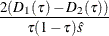where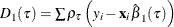is the sum of check losses for the reduced model,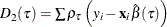is the sum of check losses for the extended model, andis the estimated sparsity function. For more information about the sparsity function, see the section Sparsity of Chapter 77: The QUANTREG Procedure.

LR2

specifies the likelihood ratio test Type II. The LR2 test score is defined as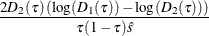whereis computed on the reduced model for testing the entry effect and on the extended model for testing the removal effect.